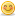1. ##i'm looking for the best way to move a ball on a
angle with velocity
I think i could use y = mx + b or somthing

eg.
angle = 90:velocity = 1
this will send the ball up slowly.
(i don't want to say ware the ball will go just the angle)

[Edited by BIacksun on 10-13-2000 at 06:46 PM]Reply With Quote

2. Code:
```vy=sin(angle)*Velocity
Vx=cos(angle)*Velocity```
well you move the ball like with a velocity vector, add the velocity component x and y to the ball coordinates.Reply With Quote

3. No that's a equation for a linear thing!
you should use something like y =x*x

or you could use a 'ballistic' equation.Reply With Quote

4. Misantrop, ballistical equations are quite too complicated for this caseReply With Quote

5. this is starting to work

Velocity = 1
ang = 90 * 3.14159
Do
X = Shape2(1).Left
y = Shape2(1).Top

X = X + Sin(ang)
y = y + Cos(ang)

Shape2(1).Left = X
Shape2(1).Top = y

For n = 1 To 1000
DoEvents
Next n
a = a + 1
Loop Until a = 1000Reply With Quote

6. wait a minute, you have to convert pi/180 degrees to get radians:
Code:
`ang = 90 * 3.14159 /180`Reply With Quote

####Posting Permissions

• You may not post new threads
• You may not post replies
• You may not post attachments
• You may not edit your posts
•